#Integrating Factor TechniqueAssume that the equation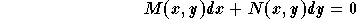,

is not exact, that is-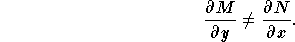In this case we look for a function u(x,y) which makes the new equation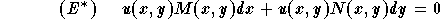,

an exact one. The function u(x,y) (if it exists) is called the integrating factor. Note that u(x,y) satisfies the following equation: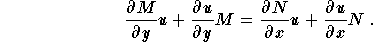This is not an ordinary differential equation since it involves more than one variable. This is what's called a partial differential equation. These types of equations are very difficult to solve, which explains why the determination of the integrating factor is extremely difficult except for the following two special cases:

Case 1: There exists an integrating factor u(x) function of x only. This happens if the expression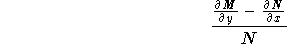,

is a function of x only, that is, the variable y disappears from the expression. In this case, the function u is given by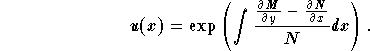Case 2: There exists an integrating factor u(y) function of y only. This happens if the expression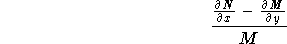,

is a function of y only, that is, the variable x disappears from the expression. In this case, the function u is given by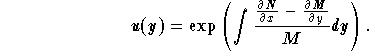Once the integrating factor is found, multiply the old equation by u to get a new one which is exact. Then you are left to use the previous technique to solve the new equation.
Advice: if you are not pressured by time, check that the new equation is in fact exact!

Let us summarize the above technique. Consider the equation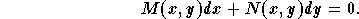If your equation is not given in this form you should rewrite it first.

Step 1: Check for exactness, that is, compute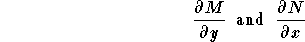,

then compare them.

Step 2: Assume that the equation is not exact (if it is exact go to step ?). Then evaluate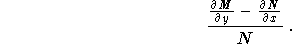If this expression is a function of x only, then go to step 3. Otherwise, evaluate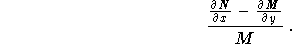If this expression is a function of y only, then go to step 3. Otherwise, you can not solve the equation using the technique developped above!

Step 3: Find the integrating factor. We have two cases:
3.1
If the expression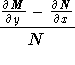is a function of x only. Then an integrating factor is given by;

3.2
If the expression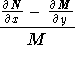is a function of y only, then an integrating factor is given byStep 4: Multiply the old equation by u, and, if you can, check that you have a new equation which is exact.

Step 5: Solve the new equation using the steps described in the previous section.

The following example illustrates the use of the integrating factor technique:[Differential Equations] [First Order D.E.]
[Geometry] [Algebra] [Trigonometry ]
[Calculus] [Complex Variables] [Matrix Algebra]S.O.S MATHematics home page

Do you need more help? Please post your question on our S.O.S. Mathematics CyberBoard.Author: Mohamed Amine Khamsi